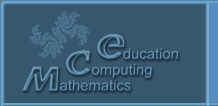Русский
 ! Вопросы и ответы

# Proceedings

## A new method of solving the differential equations by the formalization of mathematical operations of the computer

Aristov V. V., Stroganov A. V.

Russia, Moscow

In the paper the solution of the differential equation is presented as a part of the series (of the powers of the step of the independent variable). Reducing the intermediate operations in the finite-difference scheme treated as a recurrent formula is used. On the basis of a general analogy with an operation of the computer the explicit form of the solution is constructed by means of the probabilistic methods. The approach is illustrated by solutions of several nonlinear equations.

© 2004 Designed by Lyceum of Informational Technologies №1533